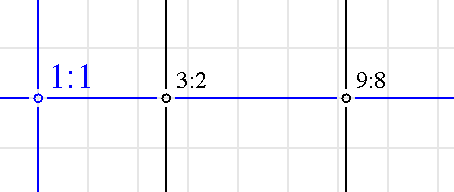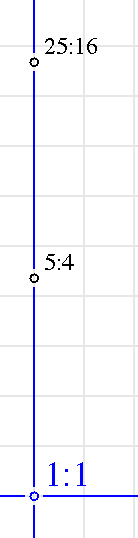Harmonic Distance Grid by Nick Didkovsky

Abstract

Harmonic Distance Grid draws a grid of Just Intonation ratios along two prime axes. The graphed distance between the ratios is proportional to the harmonic distance between them. Clicking on a ratio plays the ratio’s frequency relative to a fundamental.

Background and motivation

Harmonic Distance of a ratio a:b is defined as HD(a:b) = log­­2(ab).

For example HD(3:2)= log­­2(6)=2.584

Another example, HD(9:8)=6.169

Giacomo Fiore’s article on James Tenney’s works for guitar (see reference) included a three-dimensional lattice diagram of just intonation ratios along three prime axes: 3, 5, and 7. Ratios along the 3-prime axes, for example, begin with 1:1, and to the ‘right’, increase by a factor of 3. Ratios to the ‘left’ of 1:1 change by dividing by 3.

For example, let’s generate the first two ratios along the 3-prime axis:

1:1, 3:1, 9:1

Each ratio above is 3 times greater than the previous.

These ratios are not in standard form however. If you multiplied any of these ratios by a fundamental frequency corresponding to 1:1, you’d get frequencies way outside an octave. We want ratios representing the same relationship, but within an octave of the fundamental so we can build scales from them. A simple technique is to multiply the denominator repeatedly by 2 (ie transposing it down an octave) until you get a ratio between 1 and 2, then reducing the fraction if necessary. That gets us:

1:1, 3:2, 9:8

… all of which fall within an 2:1 octave of 1:1

Back to the lattice diagram in Fiore's paper… the nodes are graphed as equidistant from each other, which was a practical decision. It made me curious though, to see what it would look like if the distance between them corresponded to the harmonic distance.  That motivated me to write this software, and see the results as the screen shot shows below:Notice that the distance from 1:1 to 3:2 is less than the distance between 3:2 and 9:8

This graph reflects the harmonic distance between the ratios.

Similarly, the vertical axis, which defaults to 5-prime, show ratios that are a factor of 5 apart, and are graphed to show relative harmonic distance:As an aside, the default axes of 3 and 5 present what is known as a “five-limit tuning”, as scales can be created selecting ratios made of powers of 2, 3, and 5.  This software allows you to explore higher prime axes 7, 11, 13, and 17.  Unfortunately it does not show more than 2 axes at a time, so you cannot explore a complete 7 limit tuning, for example, which would require a 3 dimensional grid.  Maybe we’ll do that some day and you’ll have a 3D lattice that you can rotate and zoom in and out of.  Would be nice.

Audio

Thanks to JSyn, a Java sound synthesis API by Phil Burk ( www.softsynth.com ), I made the ratios ‘clickable’ so you can hear them.

Ex. 1:

Play a just-intonation major triad by clicking the ratios in the following sequence:

1:1, 5:4, 3:2

Ex. 2:

Try playing a just-intoned major scale on the grid by clicking the ratios in the following sequence:

1:1, 9:8, 5:4, 4:3, 3:2, 5:3, 15:8

Ex. 3:

Try following major thirds up until you get to the root again.  You’ll hear discrepancy between start and end frequencies. Click the following sequence (you’ll have to use the zoom scroll bar to zoom out a little):

1:1, 5:4, 25:16, 125:64

Now click the middle two ratios to turn them off, and you are left with something that would be a 2:1 octave in equal temperament, but instead is a little off (64 is not exactly half of 125).

Ex. 4:

Follow fifths until you get to the “Wolf Tone”. Click 1:1 and every ratio to the right of it until to get to 531441:524288 . Turn off the intermediate ratios by clicking on them and you’ll be left with two frequencies beating against each other. The fundamental at 261.626 Hz and the result of following just fifths: 265.1954, a difference of about 41 cents

Thanks:

Thanks to Giacomo Fiore and Larry Polansky for clarifying some questions about harmonic distance.  Thanks to Phil Burk for creating JSyn.

References:

Tuning Theory and Practice in James Tenney’s Works for Guitar

Giacomo Fiore

Music Theory Spectrum, mty022, https://doi.org/10.1093/mts/mty022

Published: 05 October 2018

Contact:

Nick Didkovsky, nick@didkovsky.com

www.didkovsky.com (some of my projects)

www.punosmusic.com/punosrecords.html (some of my music)

www.algomusic.com (Java Music Specification Language)